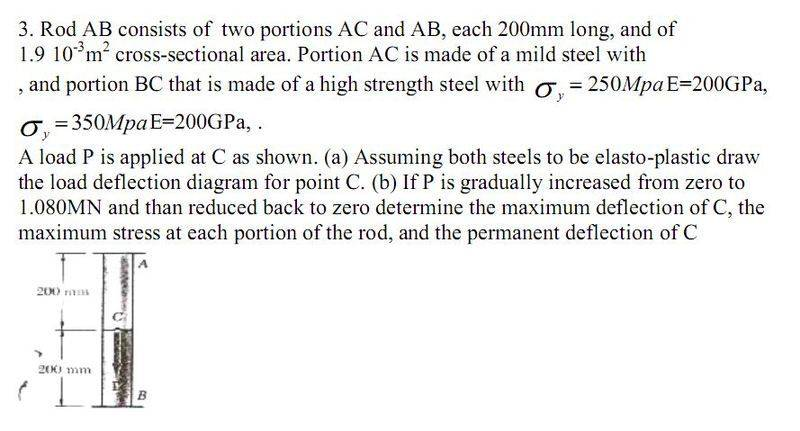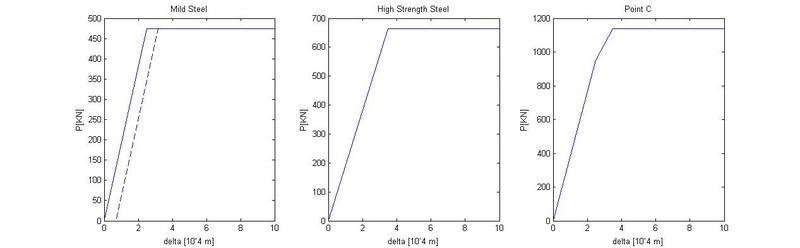in the following question, i am dealing with steel which is assumed to be have elasto-plactic behaviour,- its stress strain graph will be linear to the yield stress with a slope of "E" and then linear with a slope of zero.i calculated and drew the P(delta) graphs for the 2 steel types and added them to get the displacement of point "c"knowing that P=1080KN and looking for the maximum displacement of C, i went to the 3rd graph and said

(1080-950)/(x-2.5)=(1140-950)/(3.5-2.5)

==> x=3.1842
===> x=0.31842mm

which is correct,

now looking for the permanent deflection of "c" i know that at x=0.31842mm mild steel will have plastic deformations but high strenght steel will not yet, so i went to the 1st graph and said
(475)/(2.5)=(475)/(3.1842-x)
==> x=0.6842
===> x=0.06842mm

but the correct answer is 0.0382mm which is half of the answer i got
what am i doing wrong??

then for the second part i need to find the maximum stress in each of the steel portions,
for the mild steel i reached the plastic region- the maximum strenght, so as far as i can see the maximum stress felt in the mild steel is the stress corresponding to the 0.31842 displacement
250MPA,
which according to the question is the right answer

for tyhe high strength steel i think the maximum stress wil be at the maximum deflection, but since this is before the yield stress,
665/3.5=x/3.1842
x=605KN
x/A=318MPa

but the correct answer is 818Mpa

now i know p=1080KN
P/A=568Mpa

and using the correct answers 250-818=-568MPa

so i know that i am in the right direction but dont know what to do,
also how is it that the stress they found in the high strength steel is more than the yield stress?